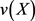# NP-PCR5融合算法及应用NP-PCR5 Fusion Algorithm and Application

DOI: 10.12677/JSTA.2019.72002, PDF, HTML, XML, 下载: 536  浏览: 1,410  国家自然科学基金支持

Abstract: This paper combines the Neutrosophic Probability theory with DSM theory, and proposes a new fusion algorithm NP-PCR5 based on the Neutrosophic Probability (NP) and Proportional Conflict Redistribution Rules (PCR). Through theoretical analysis, formula derivation and comparative experiments, the feasibility and effectiveness of the NP-PCR5 fusion algorithm in multi-sensor target recognition are proved.

1. 引言

2. 中智学

2.1. 中智逻辑

1) 不确定是由明确的不确定赋值来表述的；

2) 真、假以及不确定是相互独立的(三个不同的赋值)；

3) 它是一个量化逻辑，意味着真、假以及不确定可以用数值来表示；

4) 这种量化需要一个高度真实的间隔，这种间隔是真实数字间隔的一般化，可提供更广的解释框架；

5) 定义了许多新的连接(Neut-A, Anti-A, ……)。

$NL\left(A\right)=\left(T\left(A\right),I\left(A\right),F\left(A\right)\right)$ (1)

2.2. 中智度量

$v:X\to {R}^{3},$

$v\left(A\right)=\left(m\left(A\right),m\left(neutA\right),m\left(antiA\right)\right)$ (2)

v是一个度量函数，满足以下两个性质：

1) $v\left(\Phi \right)=\left(0,0,0\right)$

2) 可数可加性：对于 $\Sigma$ 中所有不相交的可数集 ${\left\{{A}_{n}\right\}}_{n\in L}$ ，有下式成立：

$v\left(\underset{n\in L}{\cup }{A}_{n}\right)=\left(\underset{n\in L}{\sum }m\left({A}_{n}\right),\underset{n\in L}{\sum }m\left(neut{A}_{n}\right),\underset{n\in L}{\sum }m\left(anti{A}_{n}\right)-\left(n-1\right)m\left(X\right)\right)$ (3)

$\underset{n\in L}{\sum }m\left(anti{A}_{n}\right)-\left(n-1\right)m\left(X\right)=m\left(X\right)-\underset{n\in L}{\sum }m\left({A}_{n}\right)=m\left(\underset{n\in L}{\cap }{A}_{n}\right)$ (4)

2.3. 中智度量与中智概率

$0\le v\left(X\right)=\left({x}_{1},{x}_{2},{x}_{3}\right)\le 3$ (5)

1) 当三个元素 ${x}_{1},{x}_{2},{x}_{3}$ 相互独立时，满足 $0\le {x}_{1}+{x}_{2}+{x}_{3}\le 3$

2) 当三个元素 ${x}_{1},{x}_{2},{x}_{3}$ 中有两个元素相互独立时，满足 $0\le {x}_{1}+{x}_{2}+{x}_{3}\le 2$

3) 当三个元素 ${x}_{1},{x}_{2},{x}_{3}$ 相关时，满足 $0\le {x}_{1}+{x}_{2}+{x}_{3}\le 1$

$v\left(X\right)=\left(m\left(X\right),m\left(neutX\right),m\left(antiX\right)\right)=\left({x}_{1},{x}_{2},{x}_{3}\right)$ 满足 ${x}_{1}\ge 0,{x}_{2}\ge 0,{x}_{3}\ge 0$${x}_{1}+{x}_{2}+{x}_{3}=1$ 时，就称此种形式的是中智度量的归一化。

$NP\left(A\right)=\left(m\left(A\right),m\left(neutA\right),m\left(antiA\right)\right)=\left(t,i,f\right)$ (6)

3. PCR5规则

PCR(成比例冲突再分配规则)是由Dezert和Smarandache提出来的  ，在DSm理论中共包含PCR1~PCR5五种规则，它们的不同主要在于冲突的比例再分配形式，其中，目前PCR5是从数学意义来讲最有效以及最精确的冲突分配方法。PCR5理论将辨识框架中的单焦元看作冲突信息的来源，把组合后的冲突信息都是按照单焦元的置信指派进行再分配。

${m}_{12}\left({X}_{i}\right)=\underset{\begin{array}{c}Y,Z\in {G}^{\Theta }\text{\hspace{0.17em}}\text{and}\text{\hspace{0.17em}}Y,Z\ne \varnothing \\ Y\cap Z={X}_{i}\end{array}}{\sum }{m}_{1}\left(Y\right)\cdot {m}_{2}\left(Z\right)$ (7)

$\begin{array}{cc}{m}_{12}\left({X}_{i}\right)+\underset{\begin{array}{l}{X}_{j}\in {G}^{\Theta }\text{\hspace{0.17em}}\text{and}\text{\hspace{0.17em}}i\ne j\\ {X}_{i}\cap {X}_{j}=\varnothing \end{array}}{\sum }\left[\frac{{m}_{1}{\left({X}_{i}\right)}^{2}{m}_{2}\left({X}_{j}\right)}{{m}_{1}\left({X}_{i}\right)+{m}_{2}\left({X}_{j}\right)}+\frac{{m}_{2}{\left({X}_{i}\right)}^{2}{m}_{1}\left({X}_{j}\right)}{{m}_{2}\left({X}_{i}\right)+{m}_{1}\left({X}_{j}\right)}\right]& {X}_{i}\in {G}^{\Theta }\text{\hspace{0.17em}}\text{and}\text{\hspace{0.17em}}{X}_{i}\ne \varnothing \\ 0& {X}_{i}=\varnothing \end{array}$ (8)

4. NP-PCR5融合算法

$\left[N{P}_{1}\oplus N{P}_{2}\right]\left(t\right)={t}_{1}{t}_{2}+\left(\frac{{t}_{1}^{2}{i}_{2}}{{t}_{1}+{i}_{2}}+\frac{{t}_{2}^{2}{i}_{1}}{{t}_{2}+{i}_{1}}\right)+\left(\frac{{t}_{1}^{2}{f}_{2}}{{t}_{1}+{f}_{2}}+\frac{{t}_{2}^{2}{f}_{1}}{{t}_{2}+{f}_{1}}\right)$ (9)

$\frac{{x}_{1}}{{t}_{1}}=\frac{{y}_{1}}{{i}_{2}}=\frac{{t}_{1}{i}_{2}}{{t}_{1}+{i}_{2}}$ (10)

${x}_{1}=\frac{{t}_{1}^{2}{i}_{2}}{{t}_{1}+{i}_{2}}$${y}_{1}=\frac{{t}_{1}{i}_{2}^{2}}{{t}_{1}+{i}_{2}}$ (11)

$\frac{{x}_{2}}{{t}_{2}}=\frac{{y}_{2}}{{i}_{1}}=\frac{{t}_{2}{i}_{1}}{{t}_{2}+{i}_{1}}$ (12)

${x}_{2}=\frac{{t}_{2}^{2}{i}_{1}}{{t}_{2}+{i}_{1}}$${y}_{2}=\frac{{t}_{2}{i}_{1}^{2}}{{t}_{2}+{i}_{1}}$ (13)

$\frac{{x}_{3}}{{t}_{1}}=\frac{{z}_{1}}{{f}_{2}}=\frac{{t}_{1}{f}_{2}}{{t}_{1}+{f}_{2}}$ (14)

${x}_{3}=\frac{{t}_{1}^{2}{f}_{2}}{{t}_{1}+{f}_{2}}$ (15)

$\frac{{x}_{4}}{{t}_{2}}=\frac{{z}_{2}}{{f}_{1}}=\frac{{t}_{2}{f}_{1}}{{t}_{2}+{f}_{1}}$ (16)

${x}_{4}=\frac{{t}_{2}^{2}{f}_{1}}{{t}_{2}+{f}_{1}}$${z}_{2}=\frac{{t}_{2}{f}_{1}^{2}}{{t}_{2}+{f}_{1}}$ (17)

$\frac{{y}_{3}}{{i}_{1}}=\frac{{z}_{3}}{{f}_{2}}=\frac{{i}_{1}{f}_{2}}{{i}_{1}+{f}_{2}}$ (18)

${y}_{3}=\frac{{i}_{1}^{2}{f}_{2}}{{i}_{1}+{f}_{2}}$${z}_{3}=\frac{{i}_{1}{f}_{2}^{2}}{{i}_{1}+{f}_{2}}$ (19)

$\frac{{y}_{4}}{{i}_{2}}=\frac{{z}_{4}}{{f}_{1}}=\frac{{i}_{2}{f}_{1}}{{i}_{2}+{f}_{1}}$ (20)

${y}_{4}=\frac{{i}_{2}^{2}{f}_{1}}{{i}_{2}+{f}_{1}}$${z}_{3}=\frac{{i}_{2}{f}_{1}^{2}}{{i}_{2}+{f}_{1}}$ (21)

$\left[N{P}_{1}\oplus N{P}_{2}\right]\left(t\right)={t}_{1}{t}_{2}+\left(\frac{{t}_{1}^{2}{i}_{2}}{{t}_{1}+{i}_{2}}+\frac{{t}_{2}^{2}{i}_{1}}{{t}_{2}+{i}_{1}}\right)+\left(\frac{{t}_{1}^{2}{f}_{2}}{{t}_{1}+{f}_{2}}+\frac{{t}_{2}^{2}{f}_{1}}{{t}_{2}+{f}_{1}}\right)$

$\left[N{P}_{1}\oplus N{P}_{2}\right]\left(i\right)={i}_{1}{i}_{2}+\left(\frac{{i}_{1}^{2}{t}_{2}}{{i}_{1}+{t}_{2}}+\frac{{i}_{2}^{2}{t}_{1}}{{i}_{2}+{t}_{1}}\right)+\left(\frac{{i}_{1}^{2}{f}_{2}}{{i}_{1}+{f}_{2}}+\frac{{i}_{2}^{2}{f}_{1}}{{i}_{2}+{f}_{1}}\right)$ (22)

$\left[N{P}_{1}\oplus N{P}_{2}\right]\left(f\right)={f}_{1}{f}_{2}+\left(\frac{{f}_{1}^{2}{t}_{2}}{{f}_{1}+{t}_{2}}+\frac{{f}_{2}^{2}{t}_{1}}{{f}_{2}+{t}_{1}}\right)+\left(\frac{{f}_{1}^{2}{i}_{2}}{{f}_{1}+{i}_{2}}+\frac{{f}_{2}^{2}{i}_{1}}{{f}_{2}+{i}_{1}}\right)$ (23)

5. 算例分析

$\left[N{P}_{1}\oplus N{P}_{2}\right]\left(t\right)=0.6\left(0.2\right)+\left(\frac{{0.6}^{2}\left(0.3\right)}{0.6+0.3}+\frac{{0.2}^{2}\left(0.1\right)}{0.2+0.1}\right)+\left(\frac{{0.6}^{2}\left(0.5\right)}{0.6+0.5}+\frac{{0.2}^{2}\left(0.3\right)}{0.2+0.3}\right)\approx 0.44$

$\left[N{P}_{1}\oplus N{P}_{2}\right]\left(i\right)=0.1\left(0.3\right)+\left(\frac{{0.1}^{2}\left(0.2\right)}{0.1+0.2}+\frac{{0.3}^{2}\left(0.6\right)}{0.3+0.6}\right)+\left(\frac{{0.1}^{2}\left(0.5\right)}{0.1+0.5}+\frac{{0.3}^{2}\left(0.3\right)}{0.3+0.3}\right)\approx 0.15$

$\left[N{P}_{1}\oplus N{P}_{2}\right]\left(f\right)=0.3\left(0.5\right)+\left(\frac{{0.3}^{2}\left(0.2\right)}{0.3+0.2}+\frac{{0.5}^{2}\left(0.6\right)}{0.5+0.6}\right)+\left(\frac{{0.3}^{2}\left(0.3\right)}{0.3+0.3}+\frac{{0.5}^{2}\left(0.1\right)}{0.5+0.1}\right)\approx 0.41$

6. 结论

  何友, 王国宏, 关欣, 等. 信息融合理论及应用[M]. 北京: 电子工业出版社, 2010.  黄心汉, 李新德, 译. DSmT理论及其在信息融合中的应用[M]. 北京: 国防工业出版社, 2011.  Smarandache, F. (1998) Neutroso-phy. Neutrosophic Probability, Set, and Logic. American Research Press, Rehoboth, USA, 105.  Vasantha Kan-dasamy, W.B. and Smarandache, F. (2003) Fuzzy Cognitive Maps and Neutrosophic Cognitive Maps. Xiquan, Phoenix, 211 p.  Smarandache, F. (2015) (t,i,f)-Neutrosophic Structures and I-Neutrosophic Structures. Neutrosophic Sets and Systems, 8, 3-10.  Smarandache, F. (2013) Introduction of Neutrosophic Measure, Neutrosophic Integral, and Neutrosophic Probability. Sytech, Craiova.  Smarandache, F. (2013) N-Valued Refined Neutrosophic Logic and its Applications in Physics. Progress in Physics, 4, 143-146.  Smarandache, F. and Dezert, J. (2005) Information Fusion Based on New Proportional Conflict Redistribution Rules. Proceedings of the 8th International Conference on Information Fusion, Philadelphia, 25-29 July 2005.